# VBA – Get Today’s Date (Current Date)

This tutorial demonstrates how to get today’s date in VBA.

There are a couple of ways to obtain today’s date in VBA code, namely using the VBA Date() Function or the VBA Now() Function.

## Date() Function

The Date() Function returns today’s date. In the example below, we assign today’s date to a variable and then display the date in the immediate window in the VBE Editor.

``````Dim dtToday as Date
dtToday = Date()
Debug.Print dtToday``````

Alternatively, we can display the date in a message box.

``````Sub TestDate
Dim dtToday as Date
dtToday = Date()
Msgbox "Today's date is " & dtToday
End Sub``````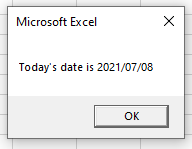## Now() Function

The Now() Function works in the same way as the Date Function, but it also includes the time.

``````Sub TestDate()
Dim dtToday As Date
dtToday = Now()
MsgBox "Today's date is " & dtToday
End Sub``````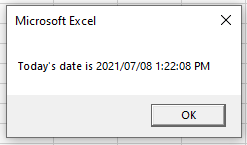## Formatting Dates With VBA

In both the Date() and the Now() Functions, the date is formatted in a default style as determined by the PC settings. We can customize this formatting using the VBA Format Function. The Format Function returns a string, so we need to declare a STRING variable rather than a DATE variable.

``````Sub TestDate()
Dim dtToday As String
dtToday = Format (Date, "dd mmmm yyyy")
MsgBox "Today's date is " & dtToday
End Sub``````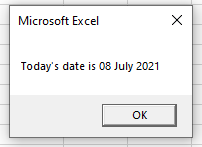We can also format the Now() Function to include the time portion in a customized format.

``````Sub FormatNow()
Dim dtToday As String
dtToday = Format(Now(), "dd mmmm yy hh:mm:ss am/pm")
MsgBox dtToday
End Sub``````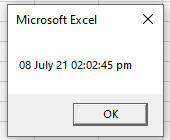## Comparing Two Dates with VBA

We can also use the Date Function to compare today’s date with a different date. For example, we might want to calculate how many days there are until an event! We can do this using the VBA DateDiff() Function which will return a number. We can therefore declare an INTEGER variable to store the returned value in.

``````Sub TestDateDiff()
Dim dtToday As Date
Dim dtSomeDay As Date
Dim iDays As Integer
dtToday = Date
dtSomeDay = "05/06/2021"
iDays = DateDiff("d", dtToday, dtSomeDay)
MsgBox "There are " & iDays & " days between the two dates"
End Sub``````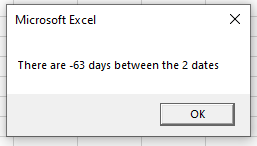As dates are stored as numbers, we could also subtract the second date from the first to obtain the same answer.

``iDays = dtToday - dtSomeDay``

Stop searching for VBA code online. Learn more about AutoMacro - A VBA Code Builder that allows beginners to code procedures from scratch with minimal coding knowledge and with many time-saving features for all users!